# JAVA 算法Bruce Duan# 二分查找

﻿

 public static int biSearch(int[] array, int a) {        int lo = 0;        int hi = array.length - 1;        int mid;        while (lo <= hi) {            // 中间位置            mid = (lo + hi) / 2;            if (array[mid] == a) {                return mid + 1;            } else if (array[mid] < a) {                // 向右查找                lo = mid + 1;            } else {                 // 向左查找                hi = mid - 1;            }        }        return -1;    }

# 冒泡排序算法

﻿

（1）比较前后相邻的二个数据，如果前面数据大于后面的数据，就将这二个数据交换。

（2）这样对数组的第 0 个数据到 N-1 个数据进行一次遍历后，最大的一个数据就“沉”到数组第

N-1 个位置。

（3）N=N-1，如果 N 不为 0 就重复前面二步，否则排序完成。

 public static void bubbleSort(int[] a, int n) {        int i, j;        for (i = 0; i < n; i++) {            // 表示 n 次排序过程。            for (j = 1; j < n - i; j++) {                // 前面的数字大于后面的数字就交换                if (a[j - 1] > a[j]) {                    // 交换 a[j-1]和 a[j]                    int temp;                    temp = a[j - 1];                    a[j - 1] = a[j];                    a[j] = temp;                }            }        }    }

# 插入排序算法

﻿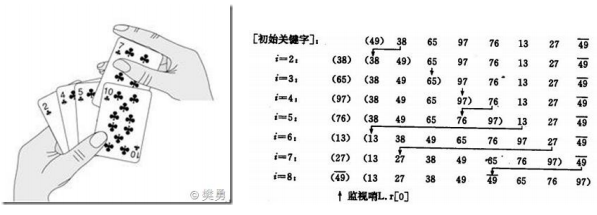﻿

public void sort(int arr[]) {        for (int i = 1; i < arr.length; i++) {            // 插入的数            int insertVal = arr[i];            // 被插入的位置(准备和前一个数比较)            int index = i - 1;            // 如果插入的数比被插入的数小            while (index >= 0 && insertVal < arr[index]) {                // 将把 arr[index] 向后移动                arr[index + 1] = arr[index];                // 让 index 向前移动                index--;            }            // 把插入的数放入合适位置            arr[index + 1] = insertVal;        }    }

# 快速排序算法

﻿

public void sort(int[] a, int low, int high) {        int start = low;        int end = high;        int key = a[low];        while (end > start) {            // 从后往前比较            while (end > start && a[end] >= key) {                // 如果没有比关键值小的，比较下一个，直到有比关键值小的交换位置，然后又从前往后比较                end--;            }            if (a[end] <= key) {                int temp = a[end];                a[end] = a[start];                a[start] = temp;            }            // 从前往后比较            while (end > start && a[start] <= key) {                // 如果没有比关键值大的，比较下一个，直到有比关键值大的交换位置                start++;            }            if (a[start] >= key) {                int temp = a[start];                a[start] = a[end];                a[end] = temp;            }            // 此时第一次循环比较结束，关键值的位置已经确定了。左边的值都比关键值小，右边的            // 值都比关键值大，但是两边的顺序还有可能是不一样的，进行下面的递归调用        }        // 递归        if (start > low) {            // 左边序列。第一个索引位置到关键值索引-1            sort(a, low, start - 1);        }        if (end < high) {            // 右边序列。从关键值索引+1 到最后一个            sort(a, end + 1, high);        }    }

﻿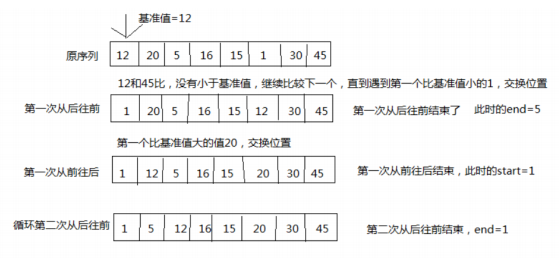# 希尔排序算法

﻿

1. 操作方法：

2. 按增量序列个数 k，对序列进行 k 趟排序；

3. 每趟排序，根据对应的增量 ti，将待排序列分割成若干长度为 m 的子序列，分别对各子表进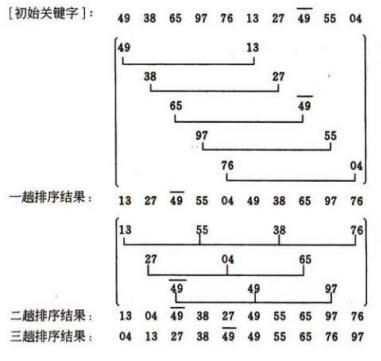﻿

private void shellSort(int[] a) {        int dk = a.length / 2;        while (dk >= 1) {            shellInsertSort(a, dk);            dk = dk / 2;        }    }    private void shellInsertSort(int[] a, int dk) {        // 类似插入排序，只是插入排序增量是 1，这里增量是 dk,把 1 换成 dk 就可以了        for (int i = dk; i < a.length; i++) {            if (a[i] < a[i - dk]) {                int j;                // x 为待插入元素                int x = a[i];                a[i] = a[i - dk];                for (j = i - dk; j >= 0 && x < a[j]; j = j - dk) {                    // 通过循环，逐个后移一位找到要插入的位置。                    a[j + dk] = a[j];                }                // 插入                a[j + dk] = x;            }        }    }

# 归并排序算法

﻿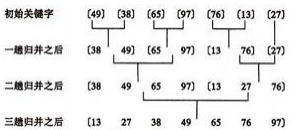﻿

public class MergeSortTest {        public static void main(String[] args) {            int[] data = new int[]{5, 3, 6, 2, 1, 9, 4, 8, 7};            print(data);            mergeSort(data);            System.out.println("排序后的数组：");            print(data);        }        public static void mergeSort(int[] data) {            sort(data, 0, data.length - 1);        }        public static void sort(int[] data, int left, int right) {            if (left >= right)                return;            // 找出中间索引             int center = (left + right) / 2;            // 对左边数组进行递归             sort(data, left, center);            // 对右边数组进行递归             sort(data, center + 1, right);            // 合并             merge(data, left, center, right);            print(data);        }        /**         * 将两个数组进行归并，归并前面 2 个数组已有序，归并后依然有序         * @param data   数组对象         * @param left   左数组的第一个元素的索引         * @param center 左数组的最后一个元素的索引，center+1 是右数组第一个元素的索引         * @param right  右数组最后一个元素的索引         */        public static void merge(int[] data, int left, int center, int right) {            // 临时数组             int[] tmpArr = new int[data.length];            // 右数组第一个元素索引             int mid = center + 1;            // third 记录临时数组的索引             int third = left;            // 缓存左数组第一个元素的索引             int tmp = left;            while (left <= center && mid <= right) {                // 从两个数组中取出最小的放入临时数组                 if (data[left] <= data[mid]) {                    tmpArr[third++] = data[left++];                } else {                    tmpArr[third++] = data[mid++];                }            }            // 剩余部分依次放入临时数组（实际上两个 while 只会执行其中一个）             while (mid <= right) {                tmpArr[third++] = data[mid++];            }            while (left <= center) {                tmpArr[third++] = data[left++];            }            // 将临时数组中的内容拷贝回原数组中             // （原 left-right 范围的内容被复制回原数组）             while (tmp <= right) {                data[tmp] = tmpArr[tmp++];            }        }        public static void print(int[] data) {            for (int i = 0; i < data.length; i++) {                System.out.print(data[i] + "\t");            }            System.out.println();        }    }

# 桶排序算法

﻿

1.找出待排序数组中的最大值 max、最小值 min

2.我们使用 动态数组 ArrayList 作为桶，桶里放的元素也用 ArrayList 存储。桶的数量为(max

min)/arr.length+1

3.遍历数组 arr，计算每个元素 arr[i] 放的桶

4.每个桶各自排序

public static void bucketSort(int[] arr) {        int max = Integer.MIN_VALUE;        int min = Integer.MAX_VALUE;        for (int i = 0; i < arr.length; i++) {            max = Math.max(max, arr[i]);            min = Math.min(min, arr[i]);        }        // 创建桶        int bucketNum = (max - min) / arr.length + 1;        ArrayList<ArrayList<Integer>> bucketArr = new ArrayList<>(bucketNum);        for (int i = 0; i < bucketNum; i++) {            bucketArr.add(new ArrayList<Integer>());        }        // 将每个元素放入桶        for (int i = 0; i < arr.length; i++) {            int num = (arr[i] - min) / (arr.length);            bucketArr.get(num).add(arr[i]);        }        // 对每个桶进行排序        for (int i = 0; i < bucketArr.size(); i++) {            Collections.sort(bucketArr.get(i));        }    }

# 基数排序算法

﻿

public class radixSort {        int[] a =        {            49, 38, 65, 97, 76, 13, 27, 49, 78, 34, 12, 64, 5, 4, 62, 99, 98, 54, 101, 56, 17, 18, 23, 34, 15, 35, 2            5, 53, 51        };        public radixSort() {            sort(a);            for (int i = 0; i < a.length; i++) {                System.out.println(a[i]);            }        }        public void sort(int[] array) {            // 首先确定排序的趟数;            int max = array;            for (int i = 1; i < array.length; i++) {                if (array[i] > max) {                    max = array[i];                }            }            int time = 0;            // 判断位数;            while (max > 0) {                max /= 10;                time++;            }            // 建立 10 个队列;            List<ArrayList> queue = new ArrayList <> ();            for (int i = 0; i < 10; i++) {                ArrayList<Integer> queue1 = new ArrayList<>();                queue.add(queue1);            }            // 进行 time 次分配和收集;            for (int i = 0; i < time; i++) {                // 分配数组元素;                for (int j = 0; j < array.length; j++) {                    // 得到数字的第 time+1 位数;                    int x = array[j] % (int) Math.pow(10, i + 1) / (int) Math.pow(10, i);                    ArrayList<Integer> queue2 = queue.get(x);                    queue2.add(array[j]);                    queue.set(x, queue2);                }                // 元素计数器                int count = 0;                // 收集队列元素                for (int k = 0; k < 10; k++) {                    while (queue.get(k).size() > 0) {                        ArrayList<Integer> queue3 = queue.get(k);                        array[count] = queue3.get(0);                        queue3.remove(0);                        count++;                    }                }            }        }    }

# 剪枝算法

﻿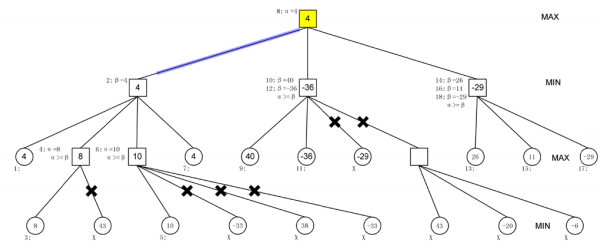﻿

﻿

# 最小生成树算法

﻿

N=(V,{E})是一个连通网，U 是顶点集 V 的一个非空子集，如果（u，v）是一条具有最小权值的边，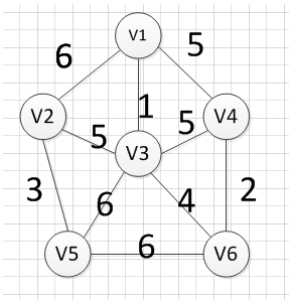﻿## 评论